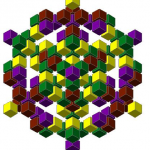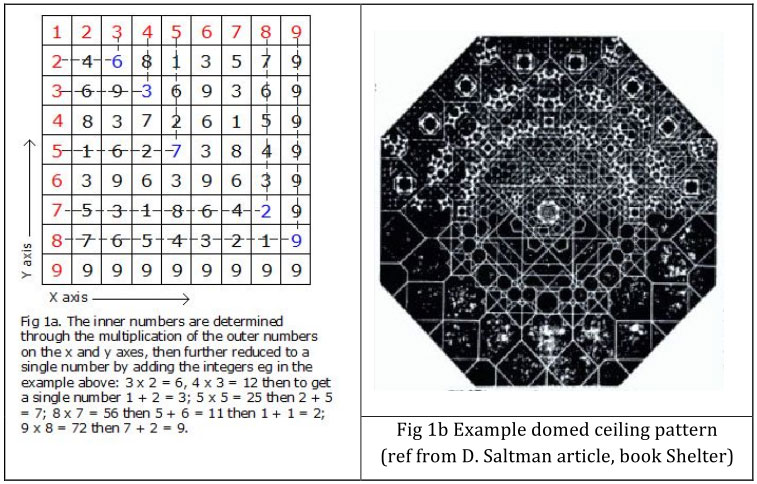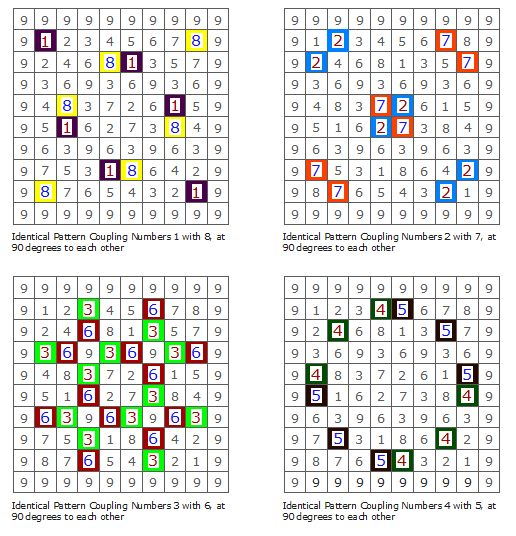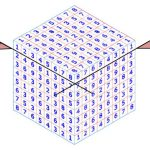### Discovered 1985 by ANTHONY JOHN WILLIAMS### Initial Inspiration.

The initial inspiration came to me in 1978 when I came across an article by David Saltman on ‘Decoding Arabic Design’. He describes the sacred ‘arabic’ Magic Number Square, passed down through time, whose origin is unclear. He mentions that the Persians linked equal numbers in this square with straight lines, shifted tracing overlays of the various patterns, and used the resulting final pattern(s) to create the designs for the complex and beautifully intricate domed and arched ceilings in their palaces and places of worship. Refer Fig 1a below to understand how the inner numbers of the square are determined by multiplication of the 1 to 9 outer numbers along the X and Y axes; if result is larger than a single number, add until reduced to single integer number.THE ARABIC MAGIC SQUARE. Note: – This is a 2D (two-dimensional) square.

I have added my own calculations to D. Saltman’s square above. I believe that the underlying mathematics shown in this two-dimensional number square is simple but profound, has been passed down through time as both useful (perhaps to ensure its survival) and sacred, and links back to a far more ancient knowledge system.

### An Astonishing Discovery.

In 1985, I discovered the hidden and inherent ‘potential’ mathematics underlying the number patterns of this sacred ‘Magic Square’. This discovery enabled an ‘unfolding’ of these number patterns downward, from flat two dimensions (2D), into the physical realm of a three-dimensional (3D) cube, made up of 1000 (10 x 10 x 10) smaller number ‘cubes’. The number positions within the Cube are not invented, but have created themselves by their own mathematical rules, as they percolate down through the layers, revealing astounding results. The following are the steps that were taken in making this discovery.

1. Amended ‘Magic Square’ I realised that the 9 by 9 magic square, starting with number 1 in the top left corner, also worked equally from the number 1 in the opposite right hand corner. Therefore, the number 9 becomes the full perimeter of a 10 x 10 square as seen below. The pattern created by number 1 is the same as created by number 8, but they are at 90 degrees to one another. Note that it is the same for numbers 2 with 7, 3 with 6 and 4 with 5. These are the four Cube number couplings, and adding both coupling numbers together = 9. So we have just four number couplings and the number 9.1. The Next Step toward Three Dimensions. (From square to cube)The ‘Magic Square’ numbers all align perfectly at the shared edges and corners if copied and fitted to the six sides of a cube. The outer perimeter of the number 9 is removed for clarity. The edge and corner numbers begin to look like small cubes. Could this top layer of numbers be sliced off and the inner numbers of the next layer down be calculated and revealed, based on the mathematics of the original ‘Magic Square’?

3. Inside the Cube. My first attempt to reveal the next layer down was unsuccessful. When I multiplied the outer edge numbers of this second layer square to determine the inner numbers as per the maths that I deduced for the first layer (i.e. the ‘Arabic Magic Square), the results were unsuccessful, and I believed then, and still do, that inner numbers by this method are probably meaningless.

4. The Eureka moment of discovery. In 1985 I re-examined my unresolved number cube from a few years before, and this time considered another mathematical method also true to the math of the original square: Not based on multiplying numbers, but by adding them. Looking at the 2D Magic Square, any outer number added to an inner number along any column results in the following number in that column. There was only one starting point where 2 x 2 = 4, but also 2 + 2 = 4; thus in the second layer 4 + 4 = 8. Suddenly, I discovered the hidden secret key, and the whole inner number arrangement fell into place within a matter of minutes. As I have discovered throughout my life, moments of insight are instantaneous, and usually occur by means of lateral thinking; the time taken even minutes after is just the time required to check this instantaneous information against the whole puzzle.

The ancient ‘Magic Square’ had then transformed itself into a 3D Cube of a thousand numbers (10x10x10). I consider this Cube to be both sacred and divine, since its nature can, I believe, be no accident of numbers, but knowledge deliberately concealed in a simple square, made sacred and useful, to be passed through time. All I have done is discover this really astounding revelation of number patterns, which penetrate through the entire Cube – from top to bottom, side to side and front to back, all numbers on all faces of the cube (turning 90 degrees on each opposite face) do not clash. The number 9 is revealed as an actual structure (the outer shell, with connecting straight inner rods in three directions from face to face) around which the other numbers weave.

The number 9 and the four number couplings revealed in this Divine or Sacred Cube reverberate through the matrix of ancient sites on Earth, as shown in the next section.

Fig 6. Within this 1000 number cube the number 9 is revealed as a ‘physical’ 3D structure i.e. 9, 9, 9 in the x, y and z axes.

In the image above, the zero and ninth levels have been left off for clarity.

5. The 10 levels of the Divine Cube (Identical from any face to opposite face of the Cube)

The first level, i.e. the outer shell or Zero level, is identical to the Tenth level, and consists only of 9’s. The Tenth level is therefore not shown separately. The outer shell of the cube, on all six faces, consists only of 9’s, connected by straight inner ‘rods’ of the number 9, as shown in the previous image.

### Three-dimensional examples of the number cube, and selected numbers within the number cube, from a 45-degree viewpoint.

These number colours are like those shown in the previous ‘levels’ of the Cube. The outer shell of nines is omitted except for part of 9’s shell shown in one image.

 Coupling numbers 1 + 8 Coupling numbers 2 + 7 Coupling numbers 3 + 6
 Coupling numbers 4 + 5 Coupling numbers 4 + 5 + 9 (part shell) All coupling numbers + 9 but excluding outer shell
 Coupling numbers 1 + 8 and 2 + 7 Inner 9’s with number 6 All coupling numbers excluding all 9’s

### Ancient and Sacred use of these numbers

The ‘Divine’ Cube is another revelation of the Ancient Codes or Matrix, the message deliberately hidden in the simple looking Persian Magic Square, and made useful enough to be passed down through history. The rigid and encompassing structure of the number 9 in the Cube, and the four pairs of coupling numbers, reverberate through the ancient world; in the ‘matrix’ location of ancient sites, the maths highlighted in the fabric of the mysterious structures, cycles of time, the movement of our planet, religious texts, legends and myths.

In terms of the Cube coupling numbers reflected in the works of the ancient world, please note: The numbers 1 and 8 are of equal pattern but at 90 degrees to one another and can be shown as 18 or 81 or 108 etc. Similarly, the numbers 2 and 7 are shown as 27 or 72; 3 and 6 as 36 or 63; 4 and 5 as 45 or 54. These coupling numbers are clearly highlighted in the ancient works as sacred and vital, as described in the many examples shown below. They also add up to and are part of the number 9, which is undoubtedly the master number behind ancient mathematics.

Please note that I have restricted the number of sources and comments in the following examples, to keep this document short.

In reference to the book, Heavens Mirror: Quest for the Lost Civilisation by Graham Hancock and Santha Faiia, there are numbers considered to be of such importance that they have been passed down in myths, legends, architecture and religious texts. The following extracts from Heaven’s Mirror are to be presented, analysed and expanded upon in relation to the Divine Cube revelation.

Firstly, regarding the mathematics by which we measure time and the universe, that have been passed through history in a variety of sacred forms:

Page 150: “The great ‘world ages’ of Hindu cosmology… Their respective durations are 1,728,000 years, 1,296,000 years, 864,000 years and 432,000… (and with respect to Angkor Wat) the key sections of the causeway 1,728 Hat, 1,296 Hat, 864 Hat and 432 Hat – the Yuga lengths scaled down by 1000… passage of time is numerically expressed by the length corresponding to yugas along the west – east axis”.

My comments: As well as the 4, 3, 2 ratio existing between these periods of time, each of these ancient number periods consist of the Cube coupling numbers by adding the number integers until a single number is reached, thus adding up to the number 9, which is what I believe to be the ‘structural number’ of our universe, i.e.:

1,728,000 years and 1,728 Hat = 1 + 7 + 2 + 8 (+0 +0 + 0) = 18 = 1 + 8 = 9;

1,296,000 years and 1,296 Hat = 1 + 2 + 9 + 6 = 18 = 1 + 8 = 9; (coupling (1+2=)3 with 6)

864,000 years and 864 Hat = 8 + 6 + 4 = 18 = 1 + 8 = 9; (coupling 8 with 1 (6+4=1+0 =)

432,000 years and 432 Hat = 4 + 3 + 2 = 9; (coupling 4 with 5 (3+2=) or (4+3=) 7 with 2)

thus making a total of 4,320,000 years, the grand total of the ‘World Ages’ of Hindu cosmology. (N.B., the ratio of the ‘world ages’ is also 4 to 3 to 2 and 4 + 3 + 2 = 9.)

At this point I would like to mention that this number 4,320,000 or its division by 10 = 432,000 or again by 10 = 43,200 is mentioned in many ancient forms such as the following:

• Kali Yuga, the last of the four world ages mentioned above, describes 1200 god years of 360 mortal years, which equals 1200 x 360 = 432,000 mortal years.
• The ancient religious Hindu texts, the Rig Veda, has 10,800 stanzas of 40 syllables each, which equals 10,800 x 40 = 432,000 syllables.
• The ratio of the scale of the Great Pyramid to our planet’s circumference of 1: 43,200 has been hinted at from ancient times. The perimeter of the base of the Great Pyramid of Giza, is to all intents and purposes 0.5 nautical miles, which when multiplied by the 43,200 ratio of the Great Pyramid equals the 21,600 nautical mile circumference of our planet

(2 + 1 + 6 = 9).

Continuing with extracts from Heavens Mirror by Graham Hancock and Santha Faiia:

Page 164: In discussing the pyramid mountain Phnom Bakheng, north of Angkor Watt, the central sanctuary is surrounded by 108 towers. “The number 108, one of the most sacred in Hindu and Buddhist cosmologies, is the sum of 72 and 36 (i.e. 72 + half of 72). As such it is a key component in the sequence of numbers linked to the earth’s axial precession… Great Cycle of 25,920 years, at the rate of one degree every 72 years.”

My comments: The Great Cycle refers to the axial precessional cycle of our planet. If one extends the longitudinal axis of our planet into space as a straight rod might, this rod would move slowly, in a circle over time. It takes approximately 72 years for our planet to move one degree around this 360-degree circle, equalling 25,920 years. For a former civilisation to know about this Great Cycle would be considered highly unlikely without highly sophisticated equipment; and yet it seems that some very early civilisations did possess this knowledge.

The sacred numbers mentioned above all add up to 9, i.e. the 108 towers, the 72 and 36 degrees, the Great or Precessional Cycle of 25,920 years = 2 + 5 + 9 + 2 + 0 = 18 = 9.

Continuing with extracts from Heavens Mirror by Graham Hancock and Santha Faiia:

Angkor Watt in Cambodia sits 72 degrees east of the Great Pyramid of Giza, and the overall major building works consists of 72 temple complexes, including Angkor Watt and Angkor Thom.

Page 184: regarding the Cambodian ancient temple complex of Angkor Thom where each of the five gates is “surmounted by four serene and gigantic sculpted faces…oriented with high precision towards the four cardinal directions” and “is approached by a bridge, and each … is lined by parallel rows of 54 devas and 54 asuras – ‘108 per avenue……altogether 540 statues’”

My comment: 72 degrees, 5 gates with 4 faces, 54 devas, 108 and 540 are the number couplings 7 with 2, 5 with 4, and 1 with 8 in the Cube and add up to the ‘structural’ number 9.

Page 194: At the exact geometric centre of enclosure of Angkor Thom is “the Bayon, 54 massive stone towers, each … is carved with four gigantic faces… making a total of 216 faces”.

My comment: 54 is the cube number coupling 5 with 4 and adds up to 9. Also 216 faces = 2 + 1 (3) + 6 = 9)

Continuing with extracts from Heavens Mirror:

Page 201, 203. “54 degrees of longitude to the east of Angkor… Nan Midol… are extensive underwater ruins”

My comment: Nan Midol is 72 degrees (number coupling 7 with 2) + 54 degrees east of the Great Pyramid of Giza = 126 degrees = (1 + 2 + 6 = 9).

Page 220: In discussing the changes to the angles of Earth’s obliquity of the ecliptic, “…the range of the obliquity cycle is 2 degrees, 24 minutes. Each degree is subdivided into 60 arc minutes… a total of 144 arc minutes… total of 8640 arc seconds “.

My comment: 144 adds up to 1 + 4 + 4 = 9, and note arc seconds 8 + 6 + 4 + 0 = 18 = 1 + 8 = 9. Again the ‘structural’ number 9 and coupling numbers are inherent in the movement of earth over time.

Page 220: “If we now divide these 8640 seconds by 40 seconds (the estimated change of obliquity in a century) we find that 216 centuries – i.e. 21,600 years – is the period required for the tilt of the Earth’s axis to shift from its minimum to its maximum value”.

My comment: It seems that there is a coincidence in the same number 21,600 applying to both the time required for the minimum to maximum shift of the tilt of the Earth’s axis and the number of nautical miles around the Earth’s axis. Also, another interesting coincidence is that the number of years required for the tilt of Earth’s axis from maximum back to maximum, minimum back to minimum, which is 43,200 (21,600 x 2) years, is the same ratio as the scale of the Great Pyramid to Earth.

Also please note that the number 20, or its multiple as 40 (seconds) above and 60 (arc minutes) above that, not itself divisible by 9, often plays a role with 9 and the other number couplings in many of these calculations, but with the result still divisible by 9. I mention this now because when looking at the DNA units of life itself, the number 20 (amino acids) is significant, as is the four coupling bases A, T, C and G.

In terms of the coupling numbers, three longitudes up to 180 degrees (half way around the world east or west from the Great Pyramid) that I do not recall being mentioned in Heaven’s Mirror are 117, 135 and 153 degrees, which combine coupling numbers 72 and 45, 63 and 54 = 117; 81 and 54, 72 and 63 = 135; 81 and 72 = 153. These longitudes may reveal important ancient sites yet to be discovered.

In terms of the latitude of these ancient sacred sites there is a hypothesis made by Keith M Hunter and various other authors like Jim Alison, that the majority of these ancient sacred sites such as Giza, Angkor, Nazca, Ollantaytambo, Easter Island etc., sit on one Great Circle around earth, tilted in a way that crosses the equator so that an equal half is above and the other half below, “Easter Island itself is a triangular shape and the three peaks form a triangle with an apex angle of 108 degrees and both base angles are 36 degrees”.

My comment: These angles reflect the Cube coupling numbers 1 with 8 and 3 with 6.

Also with reference to their website: “Ollantaytambo is 108 degrees along the Great Circle from Giza, and the azimuth from the axis point to Giza is 9 degrees and to Ollantaytambo 117 degrees”. Thus regarding the Cube coupling numbers in a longer string of numbers, for example (1 + 1 =) 2 + 7 = 9.

If the Great Circle hypotheses are true, then the coupling numbers of the number cube are reflected only in the longitudinal positioning of these mysterious ancient sites, while the latitude may be determined by their location on the Great Circle. As an aside, does another such Great Circle exist, picking up other ‘sacred’ sites?

According to the author Ralph Ellis, the one possible Hall of Records site may exist at or near K2. Ralph calculated a large triangle on the Earth’s surface, from the angles of the ‘air’ shafts in the Great Pyramid, within which K2 lies. From his positioning of this triangle, I resolved the dead centre of this triangle to be 45 degrees east of the Great Pyramid and 36 degrees north of the equator, i.e., Cube number couplings in both longitude and latitude. Both coordinates separately and together make to the structural number 9! In my opinion this rings an important bell, and possibly points to a site of great importance.

## Biblical Relevance of 999

Perhaps the relevance of the number 9 in a three-dimensional x, y, z expression, as shown in the Cube by 9, 9, 9 was considered so important to mankind’s understanding of mathematics – a pure and timeless language of the universe – that it was given prime importance in religion, legend and the physical world of structures: i.e., 999 is the recognised and popularised number for God or the Omnipotent One.

## The Mesoamerican Long Count calendar

The ‘Mayan’ Long Count Calendar (N.B. it is considered likely that this calendar predates the Mayan period) consists of precisely 1,872,000 solar days, which is 13 Baktun periods of 144,000 days. These coupling numbers 1 + 8 + 7 + 2 add up to 18, which again add up to the structural 9, as does 1+ 4 (5) + 4 = 9. The ‘Ancients’ have ensured that the significance of the number 9 permeate history. Of further interest is that the numbers within the ‘mythical’ Creation date of 3114 BCE add up to 9. This is however likely to be a coincidence unless the year 0, related to Christ, was pre-ordained.

### Linking the Cube with pi

The more detailed calculations for pi provide a seemingly never-repeated sequence of numbers. However, in terms of the very basic understanding of pi being 22 divided by 7, as used in ancient structures, pi = 3. 142857 142857… recurring. The 3 is the whole number, before the decimal point. After the decimal point, the number 1 pairs with the fourth number 8, followed by 4 which pairs with its fourth number 5, followed by 2 which pairs with 7. These are number couplings of the Cube. The numbers 3, 6 and 9 are the most frequent in the Cube and occur only on the whole number side of pi, even with 2 x Pi or 3 x pi etc. The number couplings, 1 with 8, 2 with 7 and 4 with 5, only occur after the decimal point and always retain the same order even when starting with a different number position. For example, 2 x pi = 6.285714 285714…

I find it very interesting that a complex number like pi, i.e. 22 divided by 7, which equals 3. 14285714.. (not a simple whole number), when multiplied by 280 (the royal cubit height of the pyramid) gives the simple whole number result of 880. The perimeter length of the base of the Great Pyramid is 1760 royal cubits. This is achieved by 2 x Pi (22/7) = 6.285714285… x the height of the pyramid (280) = 1760.

Sections I’ve investigated but not included in this document include:

• Interesting math regarding the missing number 0
• Any Interesting links to the structure of DNA and RNA?

To contact the author Anthony Williams, my email address is [email protected] and my website link is http://www.ideasofant.com/

## References:

Kahn L (Edited by), Shelter (1973), published by Shelter Publications, CA, USA.

Saltman D, Decoding Arabic Design – reference within Shelter (1973) listed above.

Hancock G & Faiia S, Heaven’s Mirror – Quest for the Lost Civilisation, (1998) Penguin, England.

Ellis R, K2, Quest of The Gods, (2002) Edfu Books, UK.

Hunter K & Alison J, Ancient World Mysteries, www.ancient-world-mysteries.com.

## One thought on “The Ant (1000 Number) Divine Cube”

1. Phil says:

Help! My brain hurts!